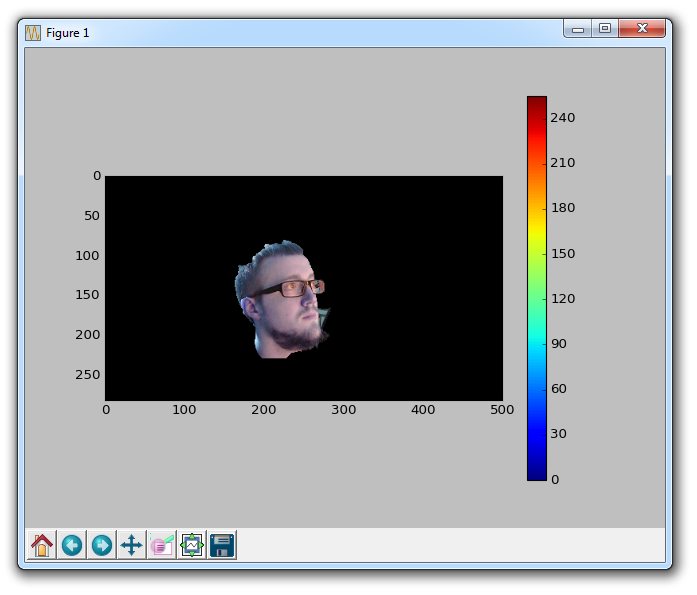## GrabCut Foreground Extraction OpenCV Python Tutorial

Welcome to a foreground extraction tutorial with OpenCV and Python. The idea here is to find the foreground, and remove the background. This is much like what a green screen does, only here we wont actually need the green screen.

To start, we will use an image:Feel free to use your own.

Let's load in the image and define a few things:

```import numpy as np
import cv2
from matplotlib import pyplot as plt

bgdModel = np.zeros((1,65),np.float64)
fgdModel = np.zeros((1,65),np.float64)

rect = (161,79,150,150)```

Thus far, we've imported cv2, numpy, and matplotlib. Then we load in the image, create a mask, specify the background and foreground model, which is used by the algorithm internally. The real important part is the rect we define. This is rect = (start_x, start_y, width, height).

This is the rectangle that encases our main object. If you're using my image, that is the rect to use. If you use your own, find the proper coordinates for your image.

Next:

```cv2.grabCut(img,mask,rect,bgdModel,fgdModel,5,cv2.GC_INIT_WITH_RECT)

plt.imshow(img)
plt.colorbar()
plt.show()```

So here we used cv2.grabCut, which took quite a few parameters. First the input image, then the mask, then the rectangle for our main object, the background model, foreground model, the amount of iterations to run, and what mode you are using.

From here, the mask is changed so that all 0 and 2 pixels are converted to the background, where the 1 and 3 pixels are now the foreground. From here, we multiply with the input image, and we get our final result:

Result:In the next tutorial, we're going to be discussing how to do corner detection.

The next tutorial:• Drawing and Writing on Image OpenCV Python Tutorial

• Image Operations OpenCV Python Tutorial

• Image arithmetics and Logic OpenCV Python Tutorial

• Thresholding OpenCV Python Tutorial

• Color Filtering OpenCV Python Tutorial

• Blurring and Smoothing OpenCV Python Tutorial

• Morphological Transformations OpenCV Python Tutorial

• Canny Edge Detection and Gradients OpenCV Python Tutorial

• Template Matching OpenCV Python Tutorial

• GrabCut Foreground Extraction OpenCV Python Tutorial
• Corner Detection OpenCV Python Tutorial

• Feature Matching (Homography) Brute Force OpenCV Python Tutorial

• MOG Background Reduction OpenCV Python Tutorial

• Haar Cascade Object Detection Face & Eye OpenCV Python Tutorial